Competitions

# Hexagonal Sticks

Time limit 1 second
Memory limit 256 MiB

In this problem, we will consider an infinite hexagonal grid. The grid consists of equal regular hexagonal cells arranged in the fashion shown below. The figure also elucidates the coordinate system used to identify each cell. Each cell of the grid can be empty or blocked.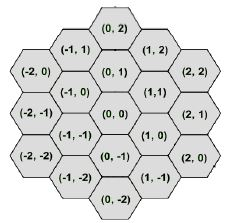Figure: A portion of the grid

There are few sticks placed randomly on this grid. The length of each stick is one 'hexagonal unit'. This means, the end points of a stick lies on the centers of neighboring cells. Your job is to move the sticks so that a closed regular hexagonal figure is formed.

The diagrams below show some closed hexagonal figures made of sticks.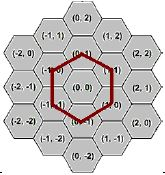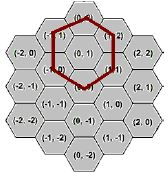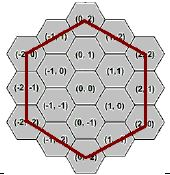Hexagon with 6 sticks Hexagon with 6 sticks Hexagon with 12 sticks

You will be given the initial coordinates of the sticks that are placed on the grid. You will also be given the coordinates of the cells that are blocked. In each move you can do one of the followings:

• Select one stick and throw it out

• Select one stick and rotate it 60 degrees clockwise/anti-clockwise about one of the end points

• Select one stick and push it along the length of the stick.

The sticks can never occupy a cell that is blocked. However, two sticks can occupy the same cells at the same time.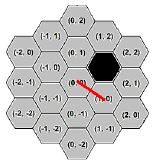Consider the scenario shown above. We have an obstacle situated at coordinate (1, 1) and a stick at coordinate (0, 0) -> (1, 0). The four possible moves that can be made are depicted below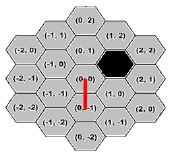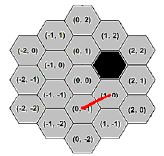Rotate 60 degrees cw about (0, 0) Rotate 60 degrees a-cw about (1, 0)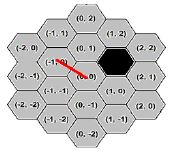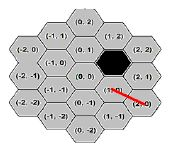Push along the length Push along the length

After all the moves are made, you have to ensure a closed regular hexagonal shape is formed with no other sticks lying around. This means the grid should contain exactly 6*x sticks where x is positive integer. Can you do this in minimum number of moves?

## Input data

The first line of input is an integer T (T < 50) that indicates the number of test cases. Each case starts with a non-negative integer S (S < 9) that gives you the number of sticks available. The next S lines give you the coordinates of the sticks. The coordinates of the sticks will be of the format x1 y1 x2 y2, which means there is a stick from (x1, y1)to (x2, y2). The coordinates will be valid and the length of each stick will be one hexagonal unit as mentioned above. The next line will give you a non-negative integer B ( B < 20) that indicates the number of obstacles. Each of the next B lines will give you the coordinates of the obstacles. The coordinate will be of the format x1 y1. It is guaranteed that the given blocks will not overlap with any given sticks. All the coordinates (sticks and blocks) will have values in the range [-4, 4].

Note: Remember that we are dealing with infinite grids. So in the optimal result, it could be possible that the hexagonal sticks lie outside [-4, 4].

## Output data

For each case, output the case number first followed by the minimum number of moves required. If it is impossible to form a hexagonal grid, output "impossible" instead. Adhere to the sample input/output for exact format.

## Examples

Input example #1
3
6
-1 -1 -1 0
-1 0 0 1
0 1 1 1
1 1 1 0
1 0 0 -1
0 -1 -1 -1
0
5
-1 0 0 1
0 1 1 1
1 1 1 0
1 0 0 -1
0 -1 -1 -1
0
7
-2 -2 -2 -1
-1 -1 -1 0
0 0 1 1
0 0 1 1
0 0 1 1
1 1 2 2
1 2 2 2
2
1 0
2 0

Output example #1
Case 1: 0
Case 2: impossible
Case 3: 9